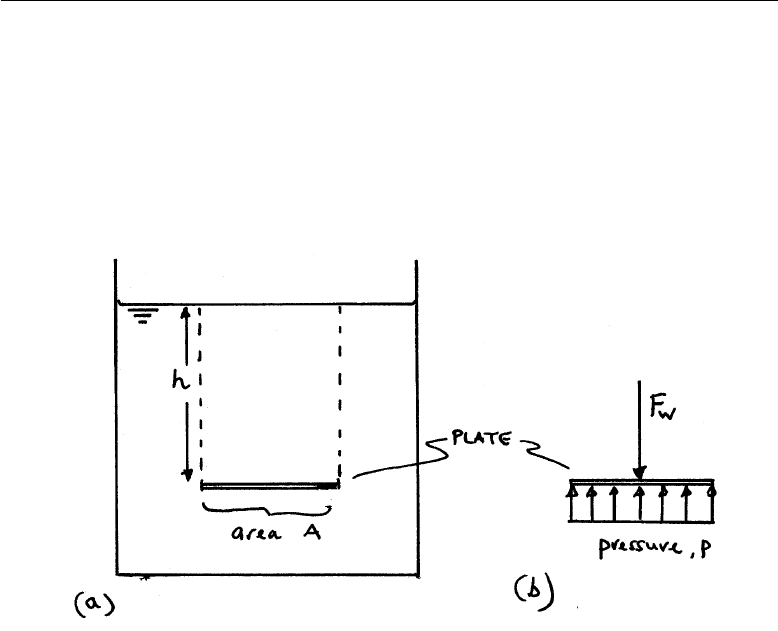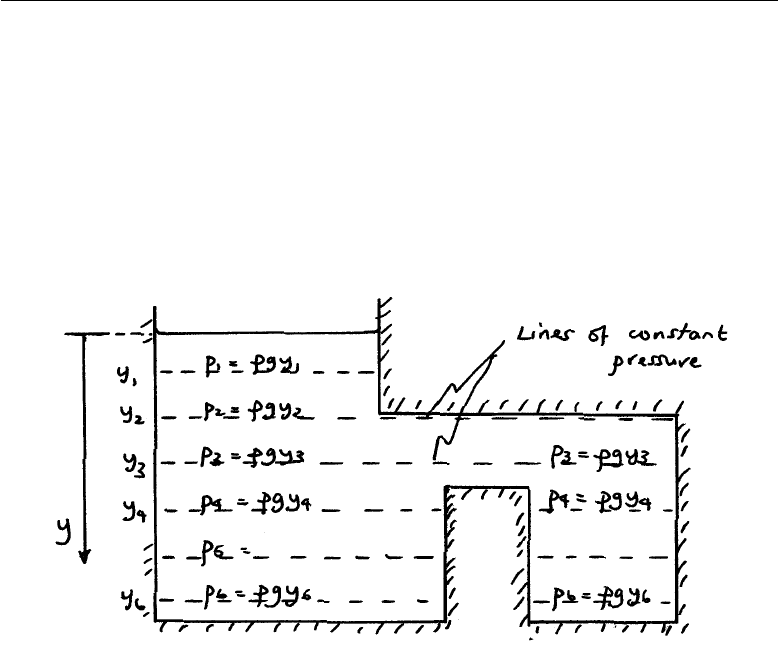# ENGR2000 Lecture Notes - Lecture 2: American Broadcasting Company, Ya Sin, Sluice

24 views18 pages
School
Department
Course
Professor2nd-Year Fluid Mechanics, Faculty of Science & Engineering, Curtin University
ENGR2000: FLUID MECHANICS
For Second-year Chemical, Petroleum, Civil & Mechanical Engineering
FLUID MECHANICS LECTURE NOTES
CHAPTER 2: HYDROSTATICS
This chapter is concerned with the eﬀect of ﬂuids when ﬂow is absent - hence
the name hydro(water1)-statics(forces balanced - hence, no acceleration). In
hydrostatics it is the gravitational forces on the bulk of ﬂuid that create the
pressure in the ﬂuid; in a later chapter we will see that ﬂuid ﬂow aﬀects this
pressure.
Hydrostatics is very important in Engineering because the hydrostatic forces
in ﬂuids can create large forces that act on structures - for example the ﬂuid
force on a dam due to the reservoir that it holds. A knowledge of these forces
then permits the appropriate design of the structure upon which they act.
We will also see that hydrostatic forces are essentially responsible for the
capability of objects, such as ships, to ﬂoat in a ﬂuid medium. (And, hot-air
balloons to ﬂoat in air.) This aspect of hydrostatics is called buoyancy and
its fundamentals are covered in this chapter.
2.1 Hydrostatic pressure
We ﬁrst wish to quantify hydrostatic pressure in a ﬂuid. We know that, for
example, a scuba diver experiences increasing pressure the deeper that s/he
dives. The simple reason for this is that the deeper s/he goes, the greater the
weight of water there is above him applying a force (per unit area) to his/her
body. This type of approach leads us to derive an expression for hydrostatic
pressure.
Consider a tank of ﬂuid which contains a very thin plate of (neutrally buoy-
ant) material with area A. This situation is shown in Fig. 2.1a. If the plate
1However, many of the principles also apply to gases - the discipline is so called because
the ﬂuid forces are very large for liquids which have a high density as compared to gases
which have relatively low densities.
Chapter 2 Page 1
find more resources at oneclass.com
find more resources at oneclass.com
Unlock document

This preview shows pages 1-3 of the document.
Unlock all 18 pages and 3 million more documents.2nd-Year Fluid Mechanics, Faculty of Science & Engineering, Curtin University
is in equilibrium (it does not start to move), then the forces on it must be
balanced. On the top side of the plate acts the weight of the ﬂuid, with den-
sity ρ, above it in the downward direction. Because it does not accelerate,
there must be a force acting upwards on the underside of the plate - this is
the eﬀect of the hydrostatic pressure acting on the plate.
FIGURE 2.1: (a) Thin plate in a ﬂuid, (b) Free-body diagram for the plate
The weight of the ﬂuid above the plate is
FW= density ×volume of ﬂuid above ×g
=ρg(Ah) (2.1)
Balancing this is the hydrostatic pressure, p, acting over the area, A, of the
underside of the plate depicted in Fig 2b. Balancing the two forces yields
pA =ρgAh
cancelling Agives the expression for the hydrostatic pressure as
p=ρgh in N/m2or Pa (Pascals) (2.2)
noting that his the vertical depth. You can now see the relationship between
hydrostatic pressure and gravitational forces. We have derived Eqn. 2.2 for
a particular depth, h. We could derive it for any depth, y, where yis a
downward coordinate from the liquid surface, giving the formula p=ρgy.
Chapter 2 Page 2
find more resources at oneclass.com
find more resources at oneclass.com
Unlock document

This preview shows pages 1-3 of the document.
Unlock all 18 pages and 3 million more documents.2nd-Year Fluid Mechanics, Faculty of Science & Engineering, Curtin University
We now note that this pressure is the same at all points in the ﬂuid with the
same vertical location (y-coordinate)) for an interconnected body of ﬂuid.
To illustrate this, lines of constant pressure are shown in Fig. 2.2.; i.e. a
plot of p=ρgy for diﬀerent values of y. The resulting diagram represents
the pressure ﬁeld in the ﬂuid. Note that these lines are all horizontal
because there is no variation with the horizontal coordinate, x, in the pressure
formula.
FIGURE 2.2: The pressure ﬁeld in an interconnected body of static ﬂuid
If we evaluated the expression above, we would not ﬁnd the total pressure
acting on the plate. This is because, using the same arguments, there is a
column of atmospheric air above the water in the tank and this presses down
on the water which, in turn, transmits the additional pressure onto the plate
. If we denote the air pressure at the liquid surface PA(again, in N/m2),
then the total pressure at a depth his
pabs =ρgh +PA(2.3)
This is called the absolute pressure whilst the pressure of Eqn. 2.2 is called
the gauge pressure, i.e. the pressure relative to atmospheric pressure.
In the derivation above, we can also see that the downward force on the
upper side of the plate also has an intensity, or pressure, given by ρgh. In
fact the eﬀect of the ﬂuid loading above results in a hydrostatic pressure,
acting downwards, on the upper side of the plate.
So far, it seems that pressure only acts in the vertical direction. We now show
that at any point in the ﬂuid, the same pressure actually acts in a direction
Chapter 2 Page 3
find more resources at oneclass.com
find more resources at oneclass.com
Unlock document

This preview shows pages 1-3 of the document.
Unlock all 18 pages and 3 million more documents.